# Gold Mine Problem

Gold Mine Problem: Here, we are going to learn about the Gold Mine Problem, its solution. This is a standard interview problem featured in coding rounds of Amazon, Flipkart.
Submitted by Radib Kar, on February 07, 2020

Description:

This is a standard interview problem featured in interview coding rounds of Amazon, Flipkart.

Problem statement:

Given a gold mine of n*m dimensions, each cell in this mine contains a positive integer which is the amount of gold in tons. Initially, the miner is at the first column but can be at any row. He can move only right or right up or right down from the current cell, i.e., the miner can move to the cell diagonally up towards the right or right or diagonally down towards the right. Find out the maximum amount of gold he can collect.

```    Input:

Matrix:
{{1,5,12},
{2,4,4},
{0,6,4}
{3,0,0}
}
Output:
18

Input:
Matrix:
{{1,3,1,5},
{2,2,4,1},
{5,0,2,3},
{0,6,11,2}
}
Output:
25
```

Explanation with example

So, the matrix is: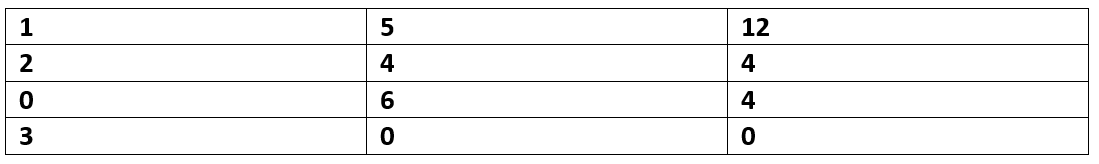So, the miner starts from the first column (any row) and he has to reach the last row with maximum gold.

To make a note, the possible moves from a cell (i,j) is to either of,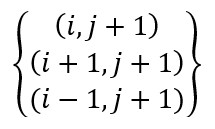Now, it seems apparently that greedy may work for the problem that is at the first column pick the cell with maximum value and then move to next best neighbouring cell.

If we follow the above greedy approach,

We would pick (3,0) as our starting cell since that contains maximum gold out of the first column. The next best move would be (2,1) and then to (2,2).

So, the total gold collected is = (3+6+4) = 13

Is this the global maximum? Do our local maximum choices lead to global maximum?

No, it's not the global maximum.

The global maximum path would be: (1,0) ->(0,1)->(0,2)

Total coin that can be achieved: (2+5+12) = 19

So, the local best choices don't lead to the global best and hence we need dynamic programming.

Solution Approach:

Create a DP matrix of dimension m*n: DP[m][n]

The first column of the DP matrix would be same as the input matrix. Rest of the columns are 0,

```    for i=0 to n-1
DP[i]=arr[i];
```

For the other columns, update DP value for every row. For every cell (i,j) update like following way.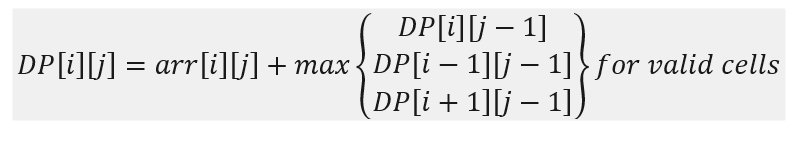So, for the earlier input matrix,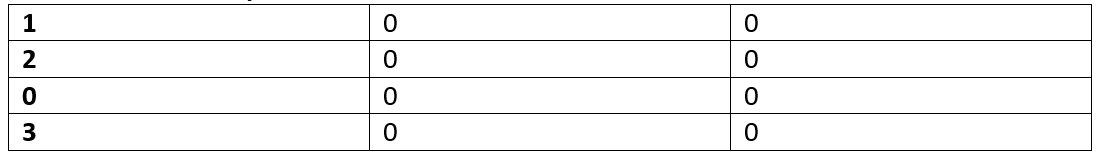After completion of the First column (row wise computing),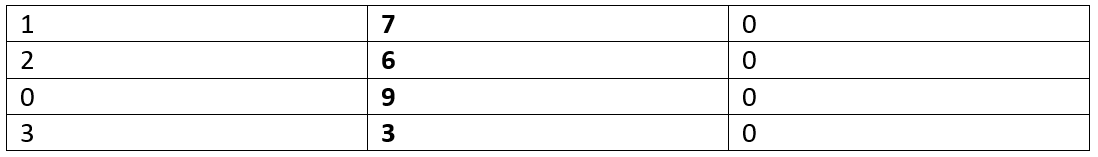After completion of second column (row wise computation),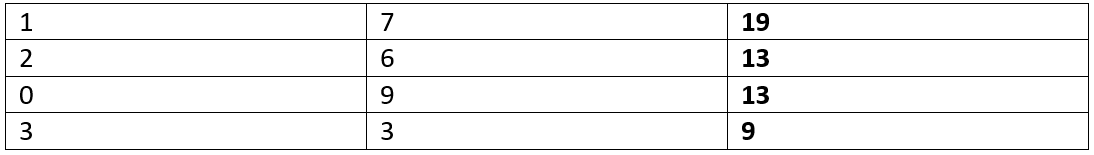Now find the maximum of the final column, that's the maximum gold that can be collected.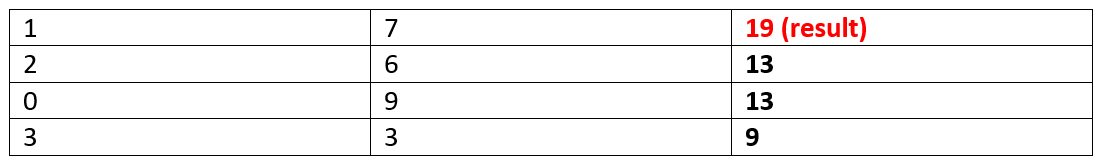Result=19

C++ implementation:

```#include <bits/stdc++.h>
using namespace std;

int GoldMine(int** arr, int n, int m)
{
//DP table
int DP[n][m];

memset(DP, 0, sizeof(DP));

for (int i = 0; i < n; i++)
DP[i] = arr[i];

//for every column
for (int j = 1; j < m; j++) {
//check which option is better accordingly
for (int i = 0; i < n; i++) {
//choosing max of possible moves
DP[i][j] = arr[i][j];
int val = DP[i][j - 1];
if (i - 1 >= 0) {
if (val < DP[i - 1][j - 1])
val = DP[i - 1][j - 1];
}
if (i + 1 < n) {
if (val < DP[i + 1][j - 1])
val = DP[i + 1][j - 1];
}
DP[i][j] += val;
}
}
// find the maximum of the last column
int gold = DP[m - 1];
for (int i = 1; i < n; i++) {
if (DP[i][m - 1] > gold)
gold = DP[i][m - 1];
}

return gold;
}

int main()
{
int n, item, m;

cout << "Enter matrix dimensions, m & n\n";
cin >> n >> m;

cout << "Input matrix cells\n";
int** arr = (int**)(malloc(sizeof(int*) * n));

//input array
for (int j = 0; j < n; j++) {
arr[j] = (int*)(malloc(sizeof(int) * m));
for (int k = 0; k < m; k++)
cin >> arr[j][k];
}

cout << "Max amount of gold that can be collected: " << GoldMine(arr, n, m) << endl;

return 0;
}
```

Output

```Enter matrix dimensions, m & n
4 3
Input matrix cells
1 5 12
2 4 4
0 6 4
3 0 0
Max amount of gold that can be collected: 19
```